# 用QGIS + OpenStreetMap + Python 處理線形地理資料

Submitted by 肥貓 on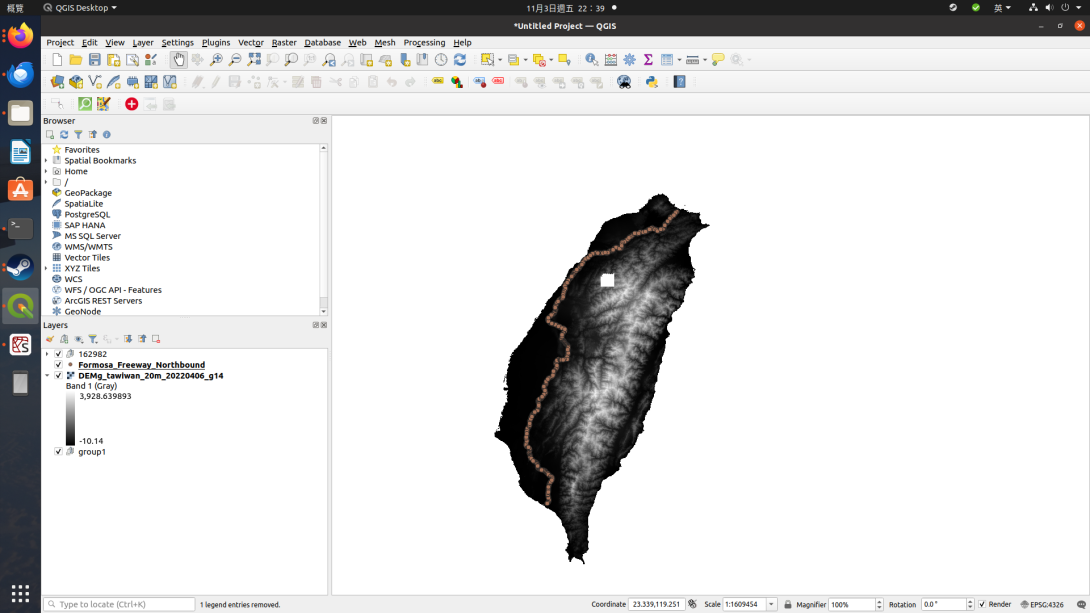## 取得圖資（XML）：

•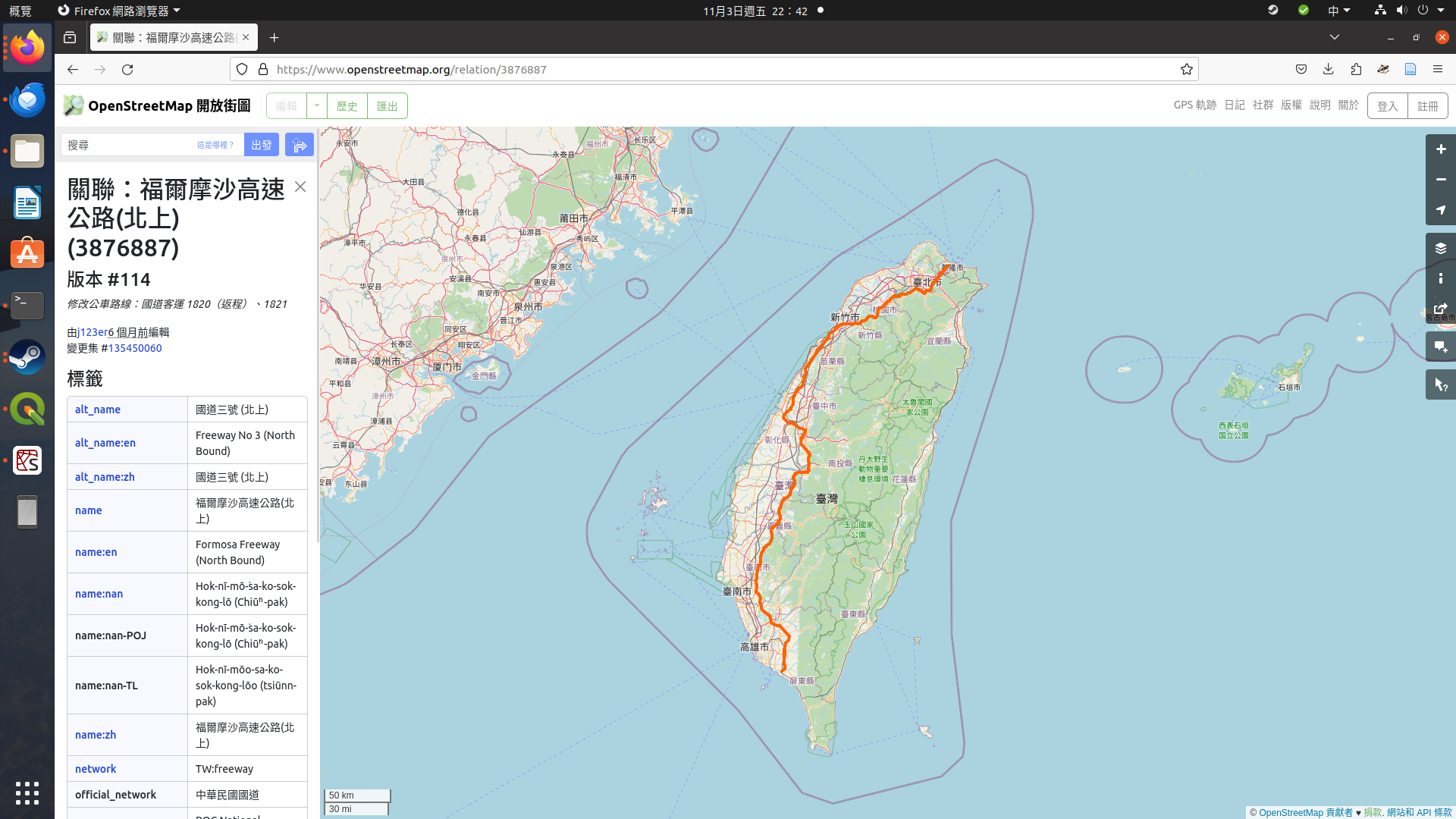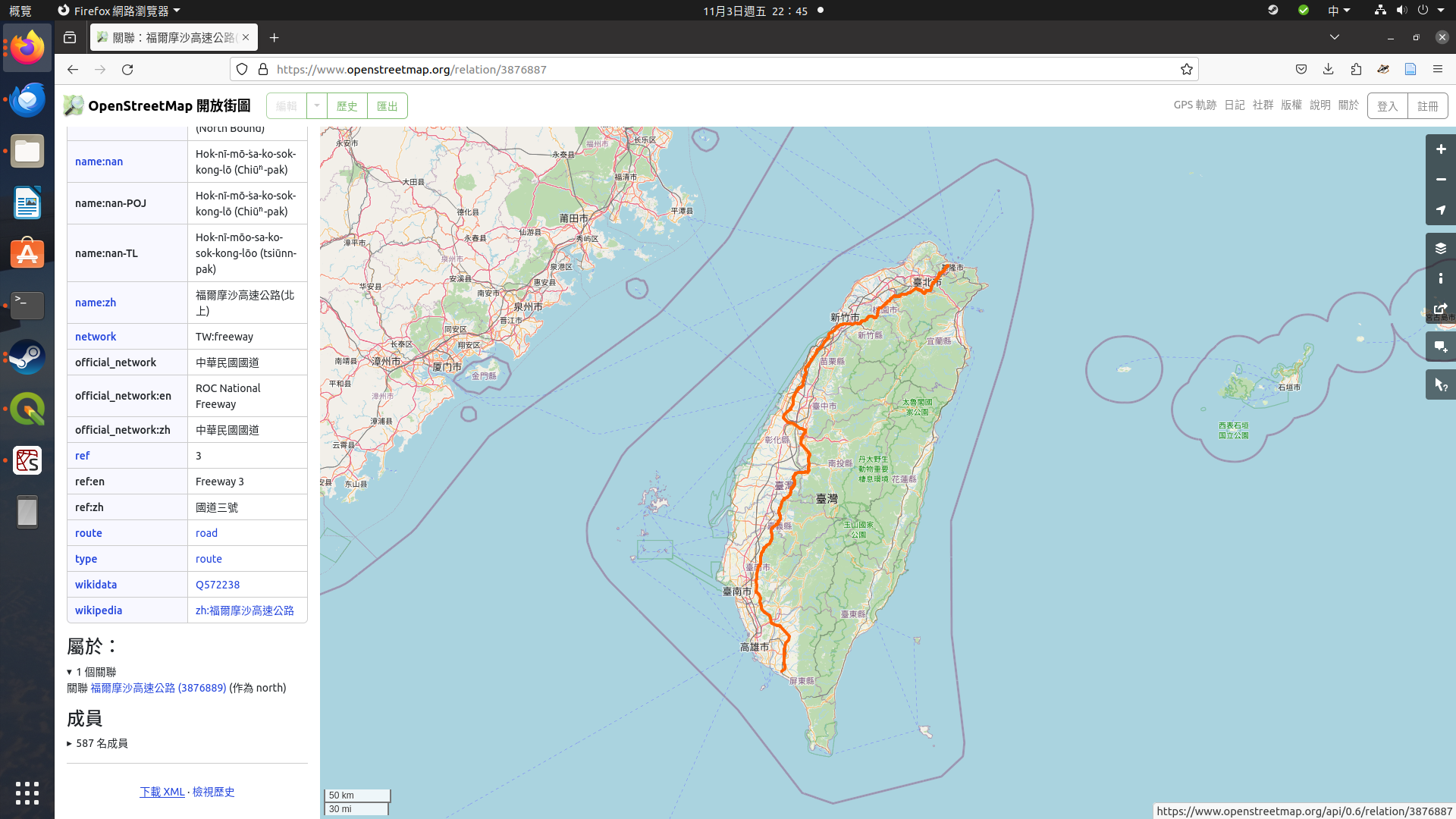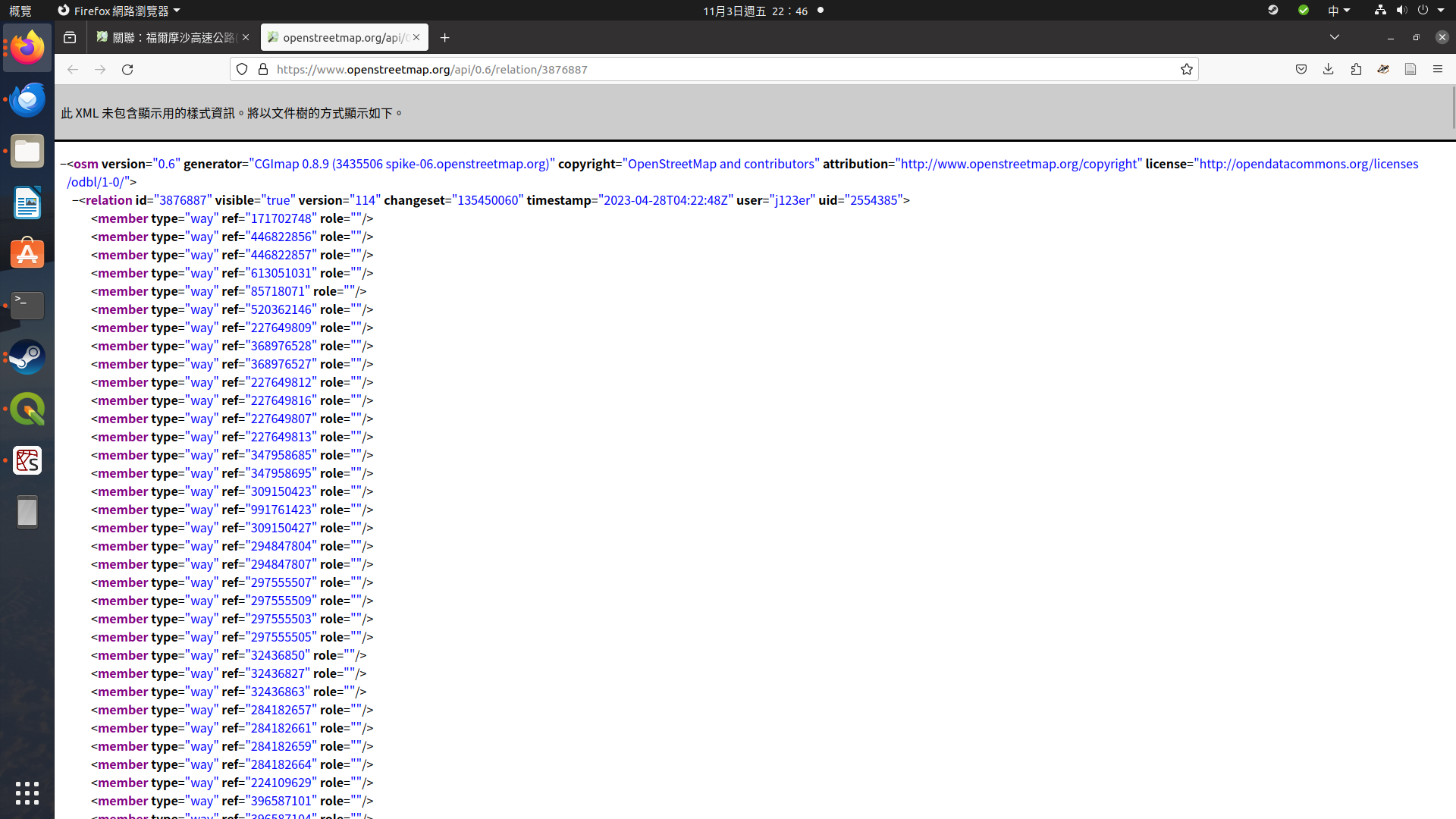https://www.openstreetmap.org/api/0.6/relation/3876887/full## 匯入Python與處理

from xml.etree import ElementTree
import pandas as pd

tree = ElementTree.parse("full.xml") #請使用您下載的檔案的位置與檔名
root = tree.getroot()

rows = []

for i in root:
rows.append(i.attrib)
cols = list(rows)
df = pd.DataFrame(rows, columns=cols)
df.to_csv('Formosa_Freeway.csv')## 將CSV檔匯入QGIS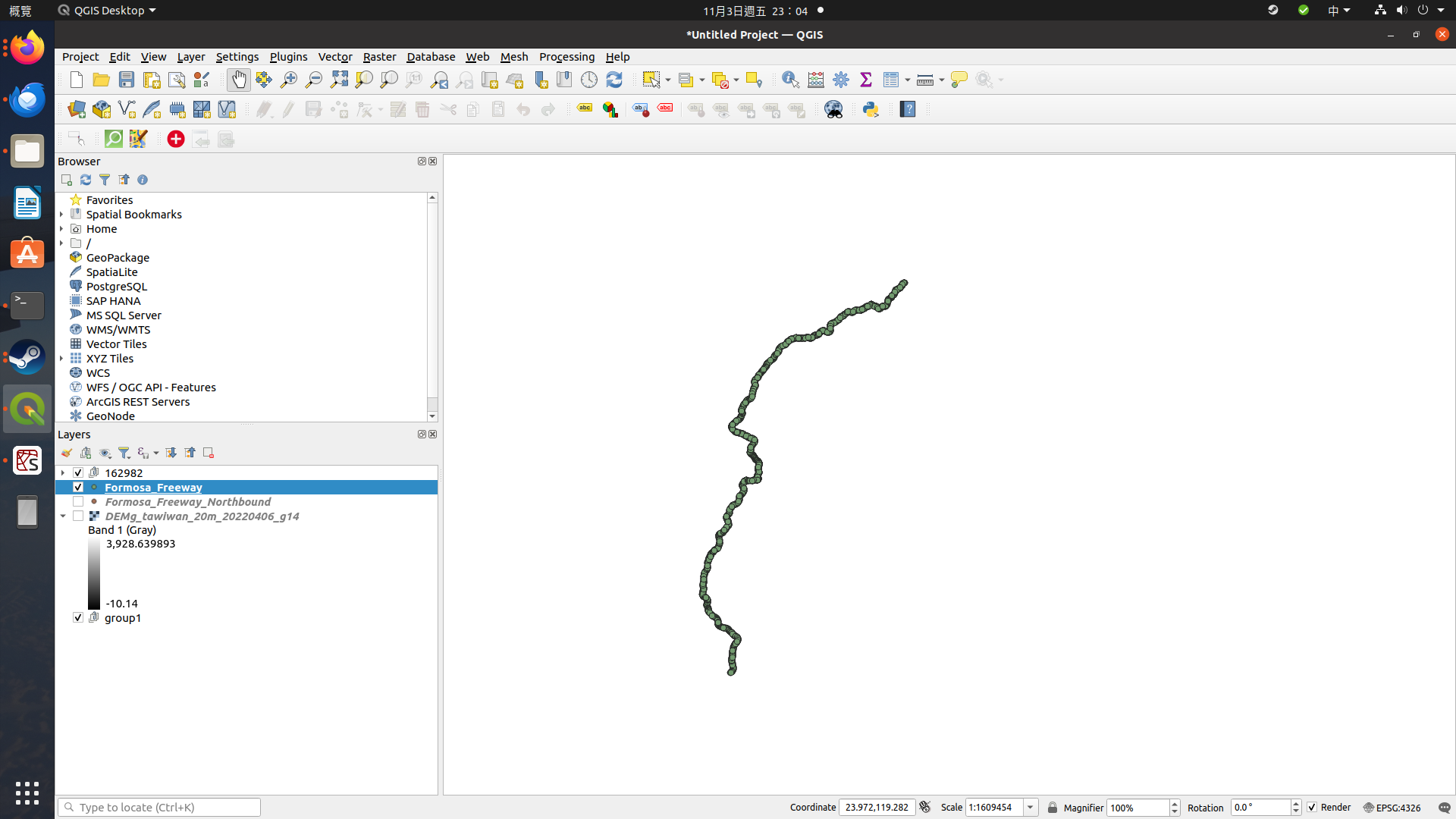## 進階利用1 - 套疊地形圖## 進階利用2：用Python自動加工OpenStreetMap的XML

import traceback
import urllib3
import xmltodict
import pandas as pd

def getxml():
url = "https://www.openstreetmap.org/api/0.6/relation/3876887/full"

http = urllib3.PoolManager()

response = http.request('GET', url)
try:
data = xmltodict.parse(response.data)
except:
print("Failed to parse xml from response (%s)" % traceback.format_exc())
return data

data=getxml()

my_list = data["osm"]["node"]
cols = list(my_list)
df = pd.DataFrame(my_list, columns=cols)
df.to_csv('Formosa_Freeway_Northbound.csv')